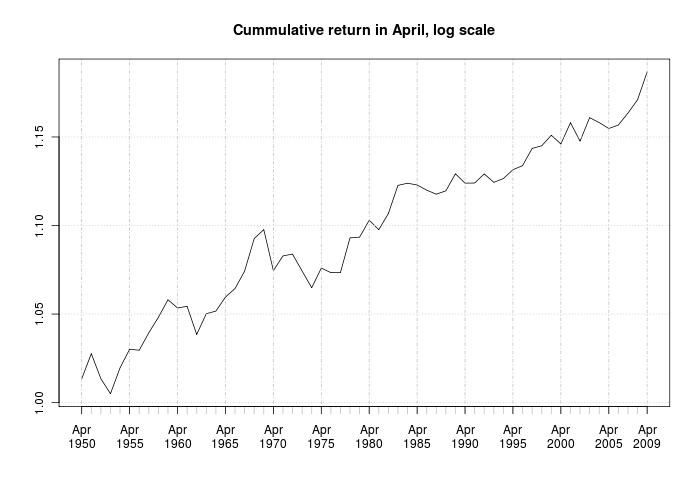Want to share your content on R-bloggers? click here if you have a blog, or here if you don't.

Bespoke blogged about average monthly returns of the DJI and emphasized April. Before jumping on that information, let’s check some weak points.
In that post, only average returns are presented. We need at least extreme points (min;max) and confidence ranges. Second problem – the normal market have upward trend and we need to get rid of that. To do so, either we have subtract the  rolling mean (a tough way) or use logarithmic prices (that’s the easy way!).

Instead of DJI, I took S&P500 from 1950 until now.Sure, average return in April is above 0, but based on historical data, negative return is possible. I conducted t-test, where null hypothesis was, that average return is equal to zero. I got p-value of 0.0042, so null hypothesis can be rejected (return is above 0).

The graph below shows cumulative return of investment, investing only in April. Keep in mind, that this is log scale and real return would be higher.Conlusion: based on this data, expect positive return in April.

R code

```require(xts)
require(quantmod)
require(ggplot2)
getSymbols('^GSPC',from='1950-01-01')
return<-Delt(Cl(to.monthly(log(GSPC))))
return<-0
temp<-as.double(format(index(return),'%m'))

temp<-data.frame(as.double(return),as.numeric(temp))
qplot(factor(as.numeric(temp[,2])),as.double(return),data=temp,geom = "boxplot",ylab='Returns',xlab='Months')

t.test(return[which(format(index(return),'%m')=='04')])
plot(cumprod(return[which(format(index(return),'%m')=='04')]+1),main='Cumulative return, 1950-present')```